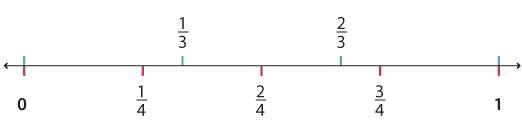### Comparing fractions on the number line

As with whole numbers, larger numbers are located to the right on a number line, and smaller numbers to the left. On the number line below we can see that 1 is larger than 0.

The number line is also useful to help us decide which of two fractions is larger. To compare $$\dfrac{2}{3} \text{and} \dfrac{3}{4}$$, we place them on the number line and see that $$\dfrac{3}{4}$$ is to the right of $$\dfrac{2}{3}$$ and so must be larger.Definition: One fraction is larger than another fraction if it lies to the right on the number line.